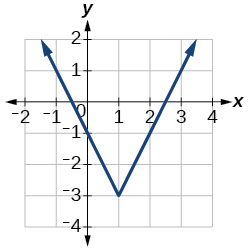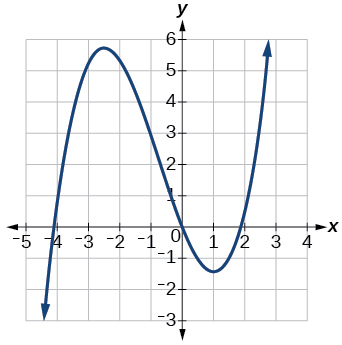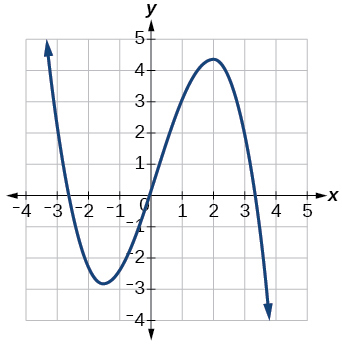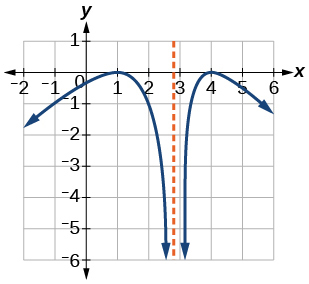# 3.3 Rates of change and behavior of graphs  (Page 5/15)

 Page 5 / 15

Access this online resource for additional instruction and practice with rates of change.

## Key equations

 Average rate of change $\frac{\Delta y}{\Delta x}=\frac{f\left({x}_{2}\right)-f\left({x}_{1}\right)}{{x}_{2}-{x}_{1}}$

## Key concepts

• A rate of change relates a change in an output quantity to a change in an input quantity. The average rate of change is determined using only the beginning and ending data. See [link] .
• Identifying points that mark the interval on a graph can be used to find the average rate of change. See [link] .
• Comparing pairs of input and output values in a table can also be used to find the average rate of change. See [link] .
• An average rate of change can also be computed by determining the function values at the endpoints of an interval described by a formula. See [link] and [link] .
• The average rate of change can sometimes be determined as an expression. See [link] .
• A function is increasing where its rate of change is positive and decreasing where its rate of change is negative. See [link] .
• A local maximum is where a function changes from increasing to decreasing and has an output value larger (more positive or less negative) than output values at neighboring input values.
• A local minimum is where the function changes from decreasing to increasing (as the input increases) and has an output value smaller (more negative or less positive) than output values at neighboring input values.
• Minima and maxima are also called extrema.
• We can find local extrema from a graph. See [link] and [link] .
• The highest and lowest points on a graph indicate the maxima and minima. See [link] .

## Verbal

Can the average rate of change of a function be constant?

Yes, the average rate of change of all linear functions is constant.

If a function $\text{\hspace{0.17em}}f\text{\hspace{0.17em}}$ is increasing on $\text{\hspace{0.17em}}\left(a,b\right)\text{\hspace{0.17em}}$ and decreasing on $\text{\hspace{0.17em}}\left(b,c\right),\text{\hspace{0.17em}}$ then what can be said about the local extremum of $\text{\hspace{0.17em}}f\text{\hspace{0.17em}}$ on $\text{\hspace{0.17em}}\left(a,c\right)?\text{\hspace{0.17em}}$

How are the absolute maximum and minimum similar to and different from the local extrema?

The absolute maximum and minimum relate to the entire graph, whereas the local extrema relate only to a specific region around an open interval.

How does the graph of the absolute value function compare to the graph of the quadratic function, $\text{\hspace{0.17em}}y={x}^{2},\text{\hspace{0.17em}}$ in terms of increasing and decreasing intervals?

## Algebraic

For the following exercises, find the average rate of change of each function on the interval specified for real numbers $\text{\hspace{0.17em}}b\text{\hspace{0.17em}}$ or $\text{\hspace{0.17em}}h$ in simplest form.

$f\left(x\right)=4{x}^{2}-7\text{\hspace{0.17em}}$ on

$4\left(b+1\right)$

$g\left(x\right)=2{x}^{2}-9\text{\hspace{0.17em}}$ on

$p\left(x\right)=3x+4\text{\hspace{0.17em}}$ on

3

$k\left(x\right)=4x-2\text{\hspace{0.17em}}$ on

$f\left(x\right)=2{x}^{2}+1\text{\hspace{0.17em}}$ on $\text{\hspace{0.17em}}\left[x,x+h\right]$

$4x+2h$

$g\left(x\right)=3{x}^{2}-2\text{\hspace{0.17em}}$ on $\text{\hspace{0.17em}}\left[x,x+h\right]$

$a\left(t\right)=\frac{1}{t+4}\text{\hspace{0.17em}}$ on $\text{\hspace{0.17em}}\left[9,9+h\right]$

$\frac{-1}{13\left(13+h\right)}$

$b\left(x\right)=\frac{1}{x+3}\text{\hspace{0.17em}}$ on $\text{\hspace{0.17em}}\left[1,1+h\right]$

$j\left(x\right)=3{x}^{3}\text{\hspace{0.17em}}$ on $\text{\hspace{0.17em}}\left[1,1+h\right]$

$3{h}^{2}+9h+9$

$r\left(t\right)=4{t}^{3}\text{\hspace{0.17em}}$ on $\text{\hspace{0.17em}}\left[2,2+h\right]$

$\frac{f\left(x+h\right)-f\left(x\right)}{h}\text{\hspace{0.17em}}$ given $\text{\hspace{0.17em}}f\left(x\right)=2{x}^{2}-3x\text{\hspace{0.17em}}$ on $\text{\hspace{0.17em}}\left[x,x+h\right]$

$4x+2h-3$

## Graphical

For the following exercises, consider the graph of $\text{\hspace{0.17em}}f\text{\hspace{0.17em}}$ shown in [link] .

Estimate the average rate of change from $\text{\hspace{0.17em}}x=1\text{\hspace{0.17em}}$ to $\text{\hspace{0.17em}}x=4.$

Estimate the average rate of change from $\text{\hspace{0.17em}}x=2\text{\hspace{0.17em}}$ to $\text{\hspace{0.17em}}x=5.$

$\frac{4}{3}$

For the following exercises, use the graph of each function to estimate the intervals on which the function is increasing or decreasing.increasing on $\text{\hspace{0.17em}}\left(-\infty ,-2.5\right)\cup \left(1,\infty \right),\text{\hspace{0.17em}}$ decreasing onincreasing on $\text{\hspace{0.17em}}\left(-\infty ,1\right)\cup \left(3,4\right),\text{\hspace{0.17em}}$ decreasing on $\text{\hspace{0.17em}}\left(1,3\right)\cup \left(4,\infty \right)$

For the following exercises, consider the graph shown in [link] .

f(x)=x/x+2 given g(x)=1+2x/1-x show that gf(x)=1+2x/3
proof
AUSTINE
sebd me some questions about anything ill solve for yall
how to solve x²=2x+8 factorization?
x=2x+8 x-2x=2x+8-2x x-2x=8 -x=8 -x/-1=8/-1 x=-8 prove: if x=-8 -8=2(-8)+8 -8=-16+8 -8=-8 (PROVEN)
Manifoldee
x=2x+8
Manifoldee
×=2x-8 minus both sides by 2x
Manifoldee
so, x-2x=2x+8-2x
Manifoldee
then cancel out 2x and -2x, cuz 2x-2x is obviously zero
Manifoldee
so it would be like this: x-2x=8
Manifoldee
then we all know that beside the variable is a number (1): (1)x-2x=8
Manifoldee
so we will going to minus that 1-2=-1
Manifoldee
so it would be -x=8
Manifoldee
so next step is to cancel out negative number beside x so we get positive x
Manifoldee
so by doing it you need to divide both side by -1 so it would be like this: (-1x/-1)=(8/-1)
Manifoldee
so -1/-1=1
Manifoldee
so x=-8
Manifoldee
Manifoldee
so we should prove it
Manifoldee
x=2x+8 x-2x=8 -x=8 x=-8 by mantu from India
mantu
lol i just saw its x²
Manifoldee
x²=2x-8 x²-2x=8 -x²=8 x²=-8 square root(x²)=square root(-8) x=sq. root(-8)
Manifoldee
I mean x²=2x+8 by factorization method
Kristof
I think x=-2 or x=4
Kristof
x= 2x+8 ×=8-2x - 2x + x = 8 - x = 8 both sides divided - 1 -×/-1 = 8/-1 × = - 8 //// from somalia
Mohamed
hii
Amit
how are you
Dorbor
well
Biswajit
can u tell me concepts
Gaurav
Find the possible value of 8.5 using moivre's theorem
which of these functions is not uniformly cintinuous on (0, 1)? sinx
which of these functions is not uniformly continuous on 0,1
solve this equation by completing the square 3x-4x-7=0
X=7
Muustapha
=7
mantu
x=7
mantu
3x-4x-7=0 -x=7 x=-7
Kr
x=-7
mantu
9x-16x-49=0 -7x=49 -x=7 x=7
mantu
what's the formula
Modress
-x=7
Modress
new member
siame
what is trigonometry
deals with circles, angles, and triangles. Usually in the form of Soh cah toa or sine, cosine, and tangent
Thomas
solve for me this equational y=2-x
what are you solving for
Alex
solve x
Rubben
you would move everything to the other side leaving x by itself. subtract 2 and divide -1.
Nikki
then I got x=-2
Rubben
it will b -y+2=x
Alex
goodness. I'm sorry. I will let Alex take the wheel.
Nikki
ouky thanks braa
Rubben
I think he drive me safe
Rubben
how to get 8 trigonometric function of tanA=0.5, given SinA=5/13? Can you help me?m
More example of algebra and trigo
What is Indices
If one side only of a triangle is given is it possible to solve for the unkown two sides?
cool
Rubben
kya
Khushnama
please I need help in maths
Okey tell me, what's your problem is?
NavinByByByByByByByBy